## 前言

Set<Person> selectAdults(Set<Person> persons) {
Set<Person> result = new HashSet<>();
for (Person person : persons)
if (person.getAge() >= 18)
return result;
}


SELECT * FROM Persons WHERE Age >= 18;


## Datalog简介

Datalog is a declarative logic programming language. While it is syntactically a subset of Prolog, Datalog generally uses a bottom-up rather than top-down evaluation model. This difference yields significantly different behavior and properties from Prolog. It is often used as a query language for deductive databases. Datalog has been applied to problems in data integration, networking, program analysis, and more.

Datalog，拆开就是Data+Logic，它有四个特点：无副作用，无控制流，无函数，非图灵完备。

### Atoms

Atoms是Datalog中的基本元素，用来以下面这种形式表示predicate：

P(X1, X2, ..., Xn)


### Datalog Rules (Logic)

H <- B1, B2, ..., Bn.


H表示head（consequent），是一个atom；B1～Bn表示body（antecedent），Bi也是atom（可以是否定的），每个Bi都是一个subgoal。上述规则的意思是：当body为真时，head为真。另外，其中的逗号,表示逻辑与，即，B1～Bn所有subgoals均为真时，body才为真，继而head才为真。例如，我们可以用如下规则推导出成年人：

Adult(person) <- Age(person, age), age >= 18


• 考虑所有subgoals中的变量的值的所有可能组合。

A(x) <- B(y), x > y.
A(x) <- B(y), !C(x, y).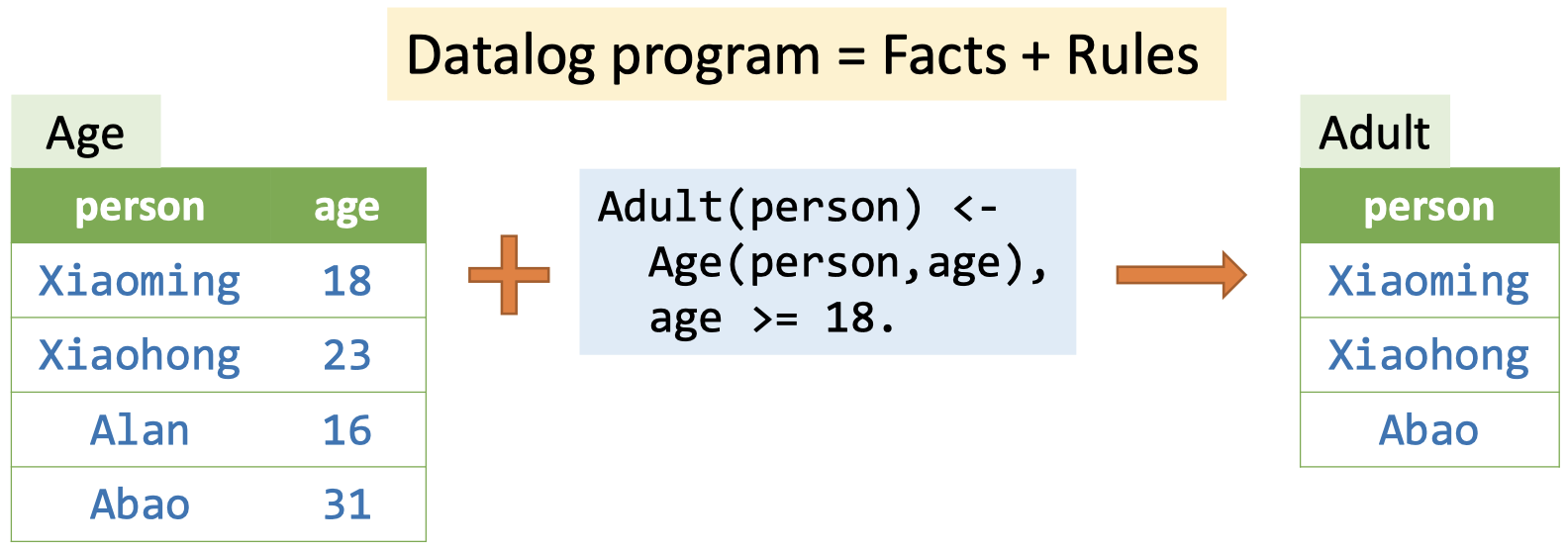### EDB和IDB谓词

Datalog中的谓词分为两部分：EDB（extensional database）和IDB（intensional database）。前者是预先定义的，作为程序输入，且不可变；后者是由规则创建的，关系由规则推理而来，可以看做程序的输出。

### 递归

Datalog支持递归的规则，允许IDB谓词的自推导。例如，我们在计算图上的可达性信息（传递闭包）时，就需要递归规则：

Reach(from, to) <- Edge(from, to).
Reach(from, to) <- Reach(from, node), Edge(node, to).


A(x) <- B(x), !A(x)


### Datalog程序的执行过程

Datalog引擎的输入是EDB和rules，输出是IDB。引擎将根据输入来推理facts，直到没有新facts产生。常见的引擎有LogicBlox、Soufflé、XSB、Datomic和Flora-2等。

## 基于Datalog的指针分析

• EDB：从程序中提取的指针相关的信息，包括：

• New(x:V, o:O)
• Assign(x:V, y:V)
• Store(x:V, f:F, y:V)
• Load(y:V, x:V, f:F)
• IDB：指针分析结果，包括：

• VarPointsTo(v : V, o : O)
• FieldPointsTo(oi : O, f : F, oj : O)

1 b = new C();
2 a = b;
3 c = new C();
4 c.f = a;
5 d = c;
6 c.f = d;
7 e = d.f;


EDB输入如下：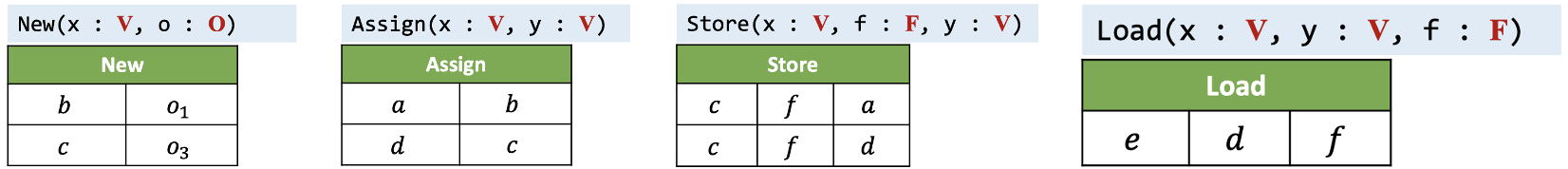New i: x = new T() $\frac{}{o_{i} \in pt(x)}$
Assign x = y $\frac{o_{i} \in pt(y)}{o_{i} \in pt(x)}$
Store x.f = y $\frac{o_{i} \in pt(x),\ o_{j} \in pt(y)}{o_{j} \in pt(o_{i}.f)}$
Load y = x.f $\frac{o_{i} \in pt(x),\ o_{j} \in pt(o_{i}.f)}{o_{j} \in pt(y)}$

VarPointsTo(x, o) <- New(x, o).
VarPointsTo(x, o) <- Assign(x, y), VarPointsTo(y, o).
FieldPointsTo(oi, f, oj) <- Store(x, f, y), VarPointsTo(x, oi), VarPointsTo(y, oj).
VarPointsTo(x, o) <- Load (y, x, f), VarPointsTo(x, oi), FieldPointsTo(oi, f, oj).


Datalog在实现上的确十分简洁。这里就不展示推理过程了，感兴趣的同学可以自行学习一下课件。

Call l: r = x.k(a1, ..., an)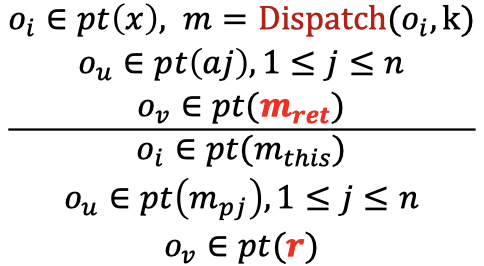Call规则比较复杂，其对应的新增EDB如下：

• VCall(l:S, x:V, k:M)
• Dispatch(o:O, k:M, m:M)
• ThisVar(m:M, this:V)
• Argument(l:S, i:N, ai:V)
• Parameter(m:M, i:N, pi:V)
• MethodReturn(m:M, ret:V)
• CallReturn(l:S, r:V)

• Reachable(m:M)
• CallGraph(l:S, m:M)

VarPointsTo(this, o), Reachable(m), CallGraph(l, m) <- VCall(l, x, k), VarPointsTo(x, o), Dispatch(o, k, m), ThisVar(m, this).
VarPointsTo(pi, o) <- CallGraph(l, m), Argument(l, i , ai), Parameter(m, i, pi), VarPointsTo(ai, o).
VarPointsTo(r, o) <- CallGraph(l, m), MethodReturn(m, ret), VarPointsTo(ret, o), CallReturn(l, r).


Reachable(m) <- EntryMethod(m).
VarPointsTo(x, o) <- Reachable(m), New(x, o, m).
VarPointsTo(x, o) <- Assign(x, y), VarPointsTo(y, o).
FieldPointsTo(oi, f, oj) <- Store(x, f, y), VarPointsTo(x, oi), VarPointsTo(y, oj).
VarPointsTo(x, o) <- Load (y, x, f), VarPointsTo(x, oi), FieldPointsTo(oi, f, oj).
VarPointsTo(this, o), Reachable(m), CallGraph(l, m) <- VCall(l, x, k), VarPointsTo(x, o), Dispatch(o, k, m), ThisVar(m, this).
VarPointsTo(pi, o) <- CallGraph(l, m), Argument(l, i , ai), Parameter(m, i, pi), VarPointsTo(ai, o).
VarPointsTo(r, o) <- CallGraph(l, m), MethodReturn(m, ret), VarPointsTo(ret, o), CallReturn(l, r).


## 基于Datalog的污点分析

• Source(m:M)
• Sink(m:M, i:N)
• Taint(l:S, t:T)

• TaintFlow(sr:S, sn:S, i:N)

Call l: r = x.k(a1, ..., an)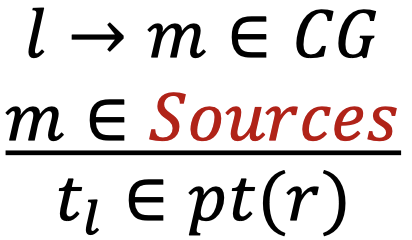Call l: r = x.k(a1, ..., an)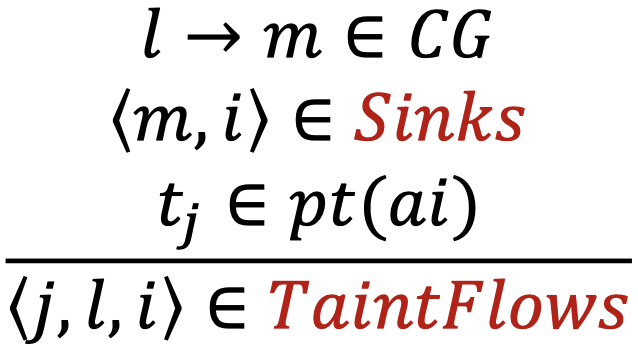VarPointsTo(r, t) <- CallGraph(l, m), Source(m), CallReturn(l, r), Taint(l, t).
TaintFlow(j, l, i) <- CallGraph(l, m), Sink(m, i), Argument(l, i, ai), VarPointsTo(ai, t), Taint(j, t).